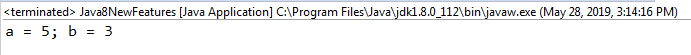• 主要介绍了使用异或实现变量互换异或加密解密示例,需要的朋友可以参考下
• 利用异或运算符实现两个数的互换 public class Tets {//借助1异或运算符实现两个数的互换 public static void main(String[] ARGS) { Scanner in=new Scanner(System.in);//创建一个Scanner对象 int n1=in....
JAVA不借助第三者实现两个变量值的互换
利用异或运算符实现两个数的互换
public class Tets {//借助1异或运算符实现两个数的互换
public static void main(String[] ARGS)
{
Scanner in=new Scanner(System.in);//创建一个Scanner对象
int n1=in.nextInt();
int n2=in.nextInt();
System.out.println("互换前第一个数为："+n1+",第二个数为："+n2);
{
n1=n1^n2;
n2=n2^n1;
n1=n1^n2;
}
System.out.println("交换后第一个数："+n1+",第二个数："+n2);
}
}


解析异或运算： a⊕b = (¬a ∧ b) ∨ (a ∧¬b)
即a=a^b;

如果a、b两个值不相同，则异或结果为1。如果a、b两个值相同，异或结果为0。例如:（同为0，异为1）
0^0=0;
0^1=1;
1^1=0;

异或运算可以使某些特定位翻转，例如在上例中输入9 7 用二进制表示：9=1001b，7=0111；
9^7=1110;
n1=9,n2=7时：
n1=9^7=1110;
n2=7^n1=0111^1110=1001=9;
n1=n1^n2=1110^1001=0111=7;互换完成

展开全文java
• 题目：int 类型的两个数，a = 3, b = 5，不借用任何变量，将a和b的值互换，并打印输出：a = 5; b = 3 答案： public static void main(String[] args) { int a = 3; int b = 5; a = a^b; b = a^b; a = a^b; ...
题目：int 类型的两个数，a = 3, b = 5，不借用任何变量，将a和b的值互换，并打印输出：a = 5; b = 3
答案：
public static void main(String[] args) {
int a = 3;
int b = 5;
a = a^b;
b = a^b;
a = a^b;
System.out.println("a = " + a + "; b = " + b);
}

运行结果：解析： 这里用到了异或运算(相同为0， 不同为1)，首先a = 3的二进制是0011；b = 5的二进制是0101; 第一步 a = a^b = 0011 ^ 0101 = 0110；这时候a = 0110，b = 0101 第二步 b = a^b = 0110 ^ 0101 = 0011；这时候a = 0110，b = 0011 第三步 a = a^b = 0110 ^ 0011 = 0101；这时候a = 0101 = 5；b = 0011 = 3展开全文• 异或运算： 异或，英文为exclusive OR，缩写成xor 异或（xor）是一个数学运算符。它应用于逻辑运算。异或的数学符号为“⊕”，计算机符号为“xor”。其运算法则为： a⊕b = (¬a ∧ b) ∨ (a ∧¬b) 如果a、b两...
异或运算：
异或，英文为exclusive OR，缩写成xor
异或（xor）是一个数学运算符。它应用于逻辑运算。异或的数学符号为“⊕”，计算机符号为“xor”。其运算法则为：
a⊕b = (¬a ∧ b) ∨ (a ∧¬b)
如果a、b两个值不相同，则异或结果为1。如果a、b两个值相同，异或结果为0。

$a = 1^ 1; //0$a = 0^ 0; //0
$a = 1^ 0; //1$a = 0^ 1; //1

示例：
数据库操作成功时返回1，而接口要求成功时error状态码为0，此时需要0和1转换，用if判断太麻烦
    public function add(Request $request) { if ($request->isPost()) {
$input =$request->param();
$opRes =$this->model->add($input); return json(['data' => '', 'error' =>$opRes['tag'] ^ 1, 'message' => \$opRes['message']]);
}
}

展开全文• 主要介绍了Java中使用异或语句实现两个变量的互换,本文直接给出代码实例以及运行结果,需要的朋友可以参考下
• 异或运算符简化两个变量值得互换
源码：

package oneday01;

 

import java.util.Scanner;

 

public class ExchangeValue {

	public static void main(String args[]) {

		Scanner scan = new Scanner(System.in);

		System.out.println("请输入A的值： ");

		long A = scan.nextLong();

		System.out.println("请输入B的值：");

		long B = scan.nextLong();

		System.out.println("A = " + A + "\tB = " + B);

		A = A ^ B;

		B = B ^ A;

		A = A ^ B;

		System.out.println("A = " + A + "\tB = " + B);

	}

}

运行结果如下：

其中下面的代码就是运用了异或运算符即  “^”：

        A = A ^ B;

		B = B ^ A;

		A = A ^ B;

		System.out.println("A = " + A + "\tB = " + B);

下面给大家详细解析一下：

1、a＝a^b

2、b＝(a^b)^b
=a^(b^b)
=
a^1
=
a

3、a=a^b
=
(a^b)^a
=
(a^a)^b
=
1
^
b=
b


展开全文• 一般情况下，如果我们要实现两个变量互换需要借助于一个第三方临时变量，这个临时变量可以临时存放某一个变量的值，帮助我们完成值的交换。有没有不需要其他变量、直接实现值互换的方法呢？ 答案是：当然有。  ...java
• 使用与运算分离奇数位与偶数位的二进制表达式，再用错位异或完成互换位运算
• 为什么用异或运算可以实现两变量的互换 文章目录为什么用异或运算可以实现两变量的互换前言一、异或运算1.基本性质2.两变量互换的关键语句二、用异或实现两变量互换 完整代码三、运算结果总结 前言 一、异或...
• ## 异或

2018-04-14 12:33:41
原文地址：https://www.lijinma.com/blog/2014/05/29/amazing-xor/ 实现A、b值互换：void swap(int &amp;a,int &amp;b){ a=a^b; b=b^a; a=a^b;}MAY 29TH, 2014什么是异或？Wikipedia的解释：在逻辑学...数学
• #include<stdio.h> #include<windows.h> #pragma warning (disable:4996) int swap( int number){ // 将 number 的二进制，奇数位与偶数位分开。 ... int n1 = number &...
• 最常用的变量互换方法是采用中间变量：void swap(int *a,int *b) { int tmp = *a; *a = *b; *b = tmp; }不用中间变量也可以实现变量互换void swap(int *a,int *b) { *a = *a + *b; *b = *a - *b; *a = *a - *b...中间变量
• 异或性质: 1. 相同为0，不同为1，即： 0^0 = 0 1^0 = 1 0^1 = 1 1^1 = 0 2. 0与其它数异或等于其自身 0^0=0 0^1=1 3. 1与其它数异或等于其取反(具有取反作用) 1^0=1 1^1=0 4. 自身与自身异或，等于将...
• 一般实现两个变量之间的互换要用第三个变量，这样做可以，但创建新变量，增加了系统开销。如果要交换的变量时两个整数型变量，可以用更高效的方法。例如:^(异或)操作，举例如下: package chapterOne; import ...
• 一般实现两个变量之间的互换要用第三个变量，这样做可以，但创建新变量，增加了系统开销。如果要交换的变量时两个整数型变量，可以用更高效的方法。例如:^(异或)操作，举例如下: import java.util.Scanner; public ...java
• C语言用异或的方法将两个数的值互换 一、简单介绍 这里介绍用异或的方法实现互换两个数的值，无需借助第3个临时变量。对于给定两个整数a,b，下面的异或运算可以实现a,b的交换：  a = a ^ b;  b = a ^ b;  a = a...
• 1，首先需要明确的是异或运算满足交换律和结合律，即有如下公式 a^b=b^a; b^a^b=a^b^b; 2,其次，异或运算还满足下面的公式 a^a=0; a^0=a; 3，使用异或运算实现两个变量交换变量值的代码如下 a=a^b;...
• ## 异或运算

万次阅读 多人点赞 2017-11-17 18:45:11
•python 算法
• ## 异或加密解密

千次阅读 2017-09-20 16:57:35
异或，英文为exclusive OR，或缩写成xor 异或（xor）是一个数学运算符。它应用于逻辑运算。异或的数学符号为“⊕”，计算机符号为“xor”。其运算法则为： a⊕b = (¬a ∧ b) ∨ (a ∧¬b) 如果a、b两个值不相同...java 加密...